SNIS189D June   2015  – June 2018

PRODUCTION DATA.

1. Features
2. Applications
3. Description
4. Revision History
5. Pin Configuration and Functions
6. Specifications
7. Detailed Description
8. Application and Implementation
1. 8.1 Application Information
2. 8.2 Typical Application
1. 8.2.1 3.3-V System VDD MSP430 Interface - Using Comparator Input
3. 8.3 System Examples
9. Power Supply Recommendations
10. 10Layout
11. 11Device and Documentation Support
12. 12Mechanical, Packaging, and Orderable Information

• DQX|2
• LPG|2
• DQX|2

### 6.10 Typical CharacteristicsUsing Electrical Characteristics - TO-92/LPG Pulse Count to Temperature LUT VP – VN = 2.15 V
Figure 2. Accuracy vs LMT01 Junction Temperature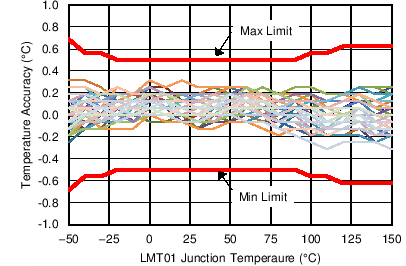Using Electrical Characteristics - TO-92/LPG Pulse Count to Temperature LUT VP – VN = 2.7 V
Figure 4. Accuracy vs LMT01 Junction Temperature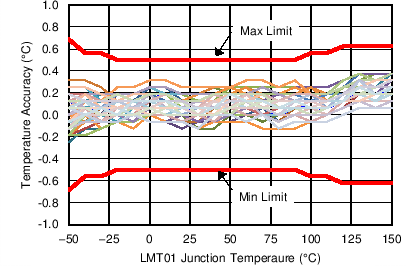Using Electrical Characteristics - TO-92/LPG Pulse Count to Temperature LUT VP – VN = 4 V
Figure 6. Accuracy vs LMT01 Junction Temperature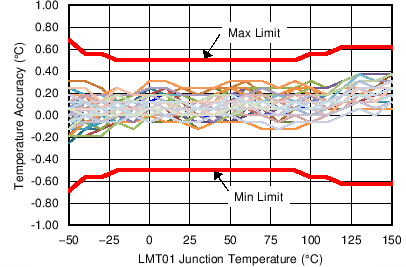Using Electrical Characteristics - TO-92/LPG Pulse Count to Temperature LUT VP – VN = 5.5 V
Figure 8. Accuracy vs LMT01 Junction Temperature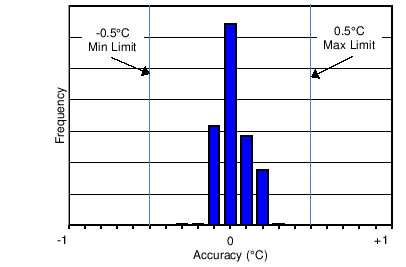Using Electrical Characteristics - TO-92/LPG Pulse Count to Temperature LUT VP – VN = 2.15 V to 5.5 V
Figure 10. Accuracy Histogram at 30°C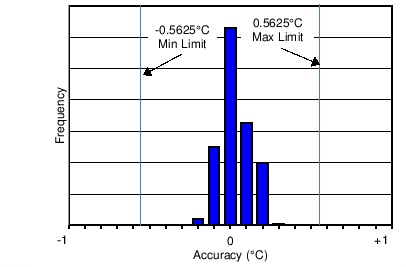Using LUT Electrical Characteristics - TO-92/LPG Pulse Count to Temperature LUT VP – VN = 2.15 V to 5.5 V
Figure 12. Accuracy Histogram at -30°C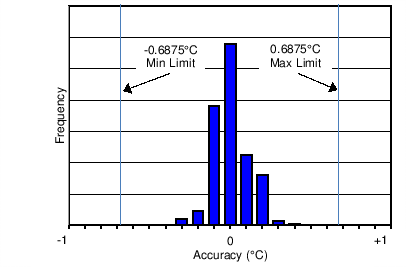Using LUT Electrical Characteristics - TO-92/LPG Pulse Count to Temperature LUT VP – VN = 2.15 V to 5.5 V
Figure 14. Accuracy Histogram at -50°C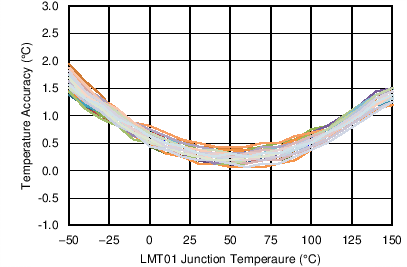Using Temp = (PC/4096 × 256°C ) – 50°C VP – VN = 5.5V
Figure 16. Accuracy Using Linear Transfer Function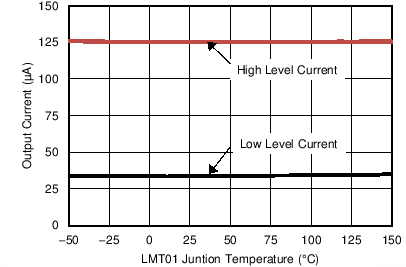VP – VN = 3.3 V
Figure 18. Output Current vs Temperature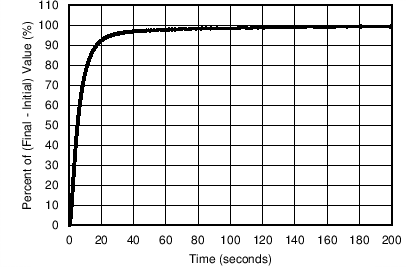VP – VN = 3.3 V Air Flow = 2.34 meters/sec TINITIAL = 23°C, TFINAL = 70°C
Figure 20. Thermal Response in Moving Air (TO92S/LPG Package)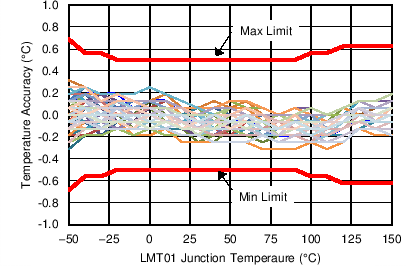Using Electrical Characteristics - TO-92/LPG Pulse Count to Temperature LUT VP – VN = 2.4 V
Figure 3. Accuracy vs LMT01 Junction Temperature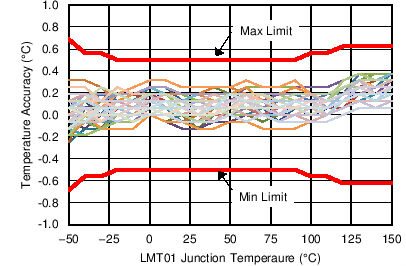Using Electrical Characteristics - TO-92/LPG Pulse Count to Temperature LUT VP – VN = 3 V
Figure 5. Accuracy vs LMT01 Junction Temperature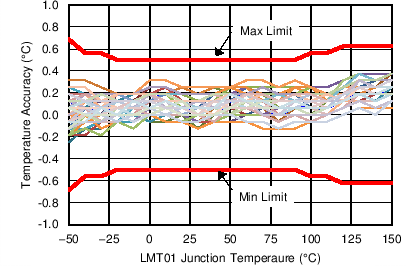Using Electrical Characteristics - TO-92/LPG Pulse Count to Temperature LUT VP – VN = 5 V
Figure 7. Accuracy vs LMT01 Junction TemperatureUsing Electrical Characteristics - TO-92/LPG Pulse Count to Temperature LUT VP – VN = 2.15 V to 5.5 V
Figure 9. Accuracy Histogram at 150°C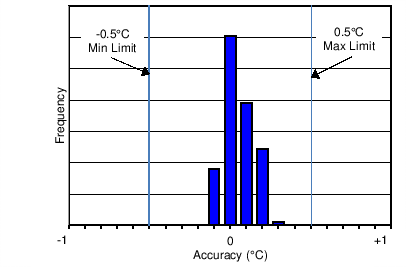Using Electrical Characteristics - TO-92/LPG Pulse Count to Temperature LUT VP – VN = 2.15 V to 5.5 V
Figure 11. Accuracy Histogram at –20°CUsing Electrical Characteristics - TO-92/LPG Pulse Count to Temperature LUT VP – VN = 2.15 V to 5.5 V
Figure 13. Accuracy Histogram at -40°C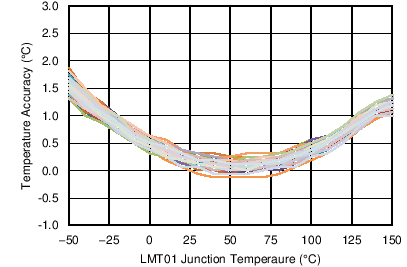Using Temp = (PC/4096 × 256°C ) – 50°C VP – VN = 2.15 V
Figure 15. Accuracy Using Linear Transfer Function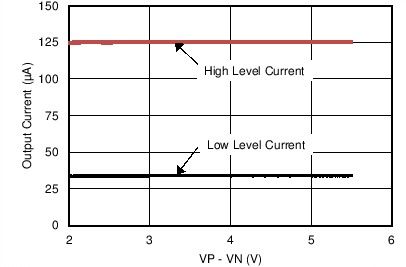TA = 30°C
Figure 17. Output Current vs VP-VN Voltage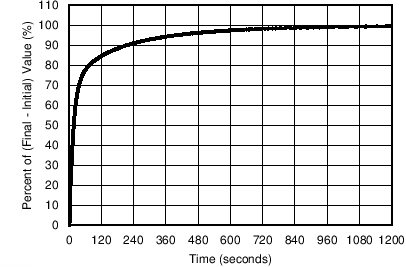VP – VN = 3.3 V TINITIAL = 23°C, TFINAL = 70°C
Figure 19. Thermal Response in Still Air (TO92S/LPG Package)VP – VN = 3.3 V TINITIAL = 23°C, TFINAL = 70°C
Figure 21. Thermal Response in Stirred Oil (TO92S/LPG Package)